#Function Repository Resource:

# HexagonalGridGraph

Generate a graph corresponding to a hexagonal grid

Contributed by: Wolfram Research
 ResourceFunction["HexagonalGridGraph"][{width,height}] returns a hexagonal grid graph with dimensions width×height.

## Details and Options

ResourceFunction["HexagonalGridGraph"] takes the same options as Graph.

## Examples

### Basic Examples (1)

A 6×6 hexagonal grid graph:

 In:=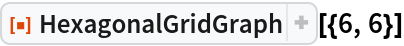Out=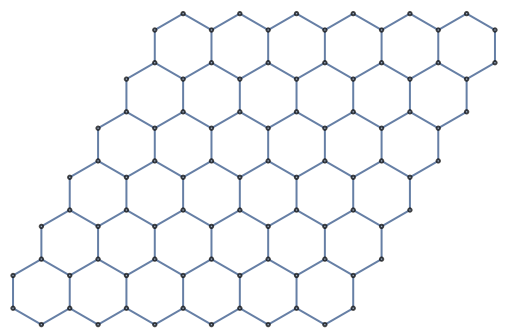### Scope (2)

Use a different graph embedding:

 In:=Out=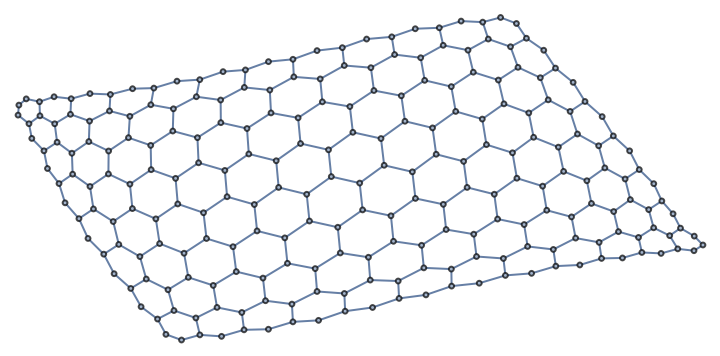Use a different plot theme:

 In:=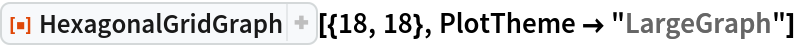Out=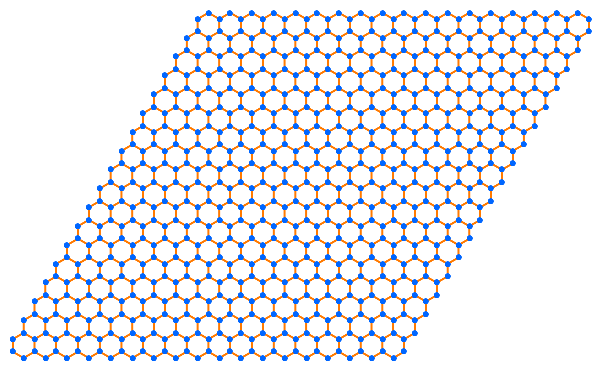### Properties and Relations (2)

Large graphs may be formatted differently in OutputForm than smaller graphs. A 50×60 hexagonal grid graph shows explicit edges and vertices:

 In:=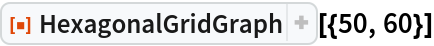Out=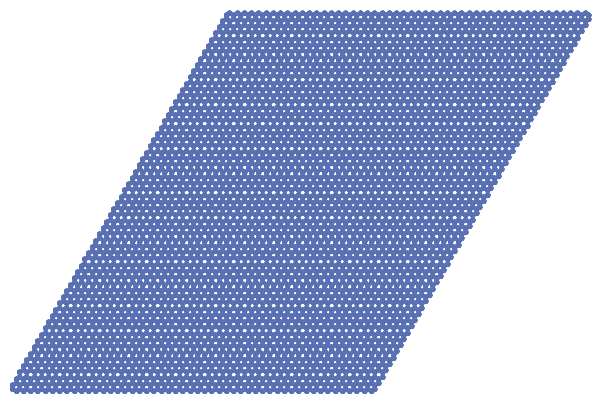A 60×60 hexagonal grid graph displays as an elided Graph expression:

 In:=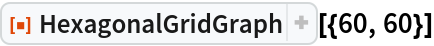Out=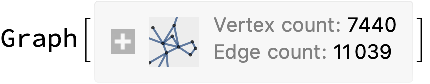## Version History

• 2.0.0 – 15 April 2020